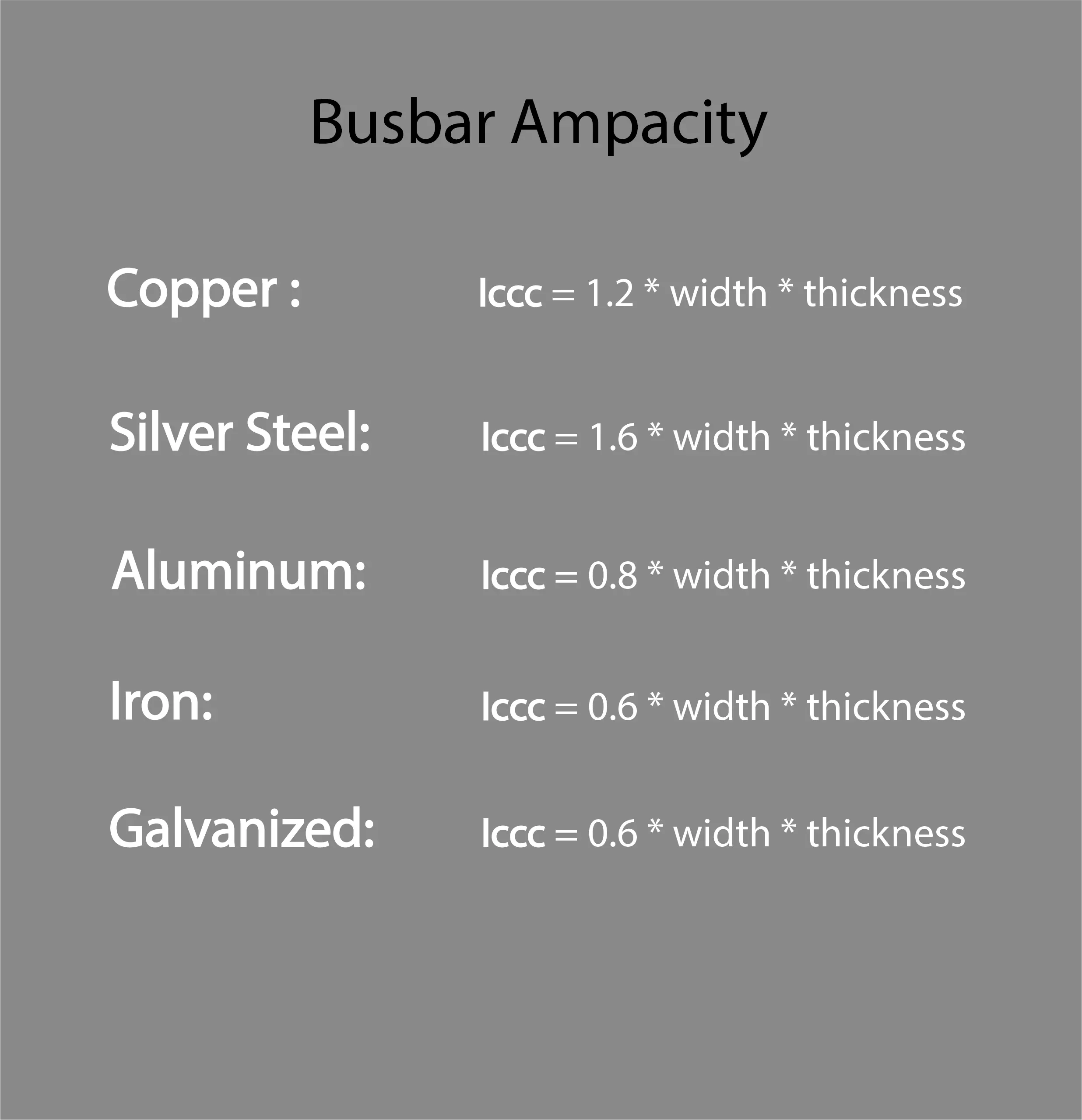(206) 687-4009 | [email protected]

# BUSBAR SIZE CALCULATOR

Electrical power system consists of multiple incoming and outgoing feeder connection, for this electrical connection busbars are required. Busbar is simply a node (conductor or group of conductors) which collects power from incoming feeder and distribute it to outgoing feeders. A busbar size is defined according to its material and current carrying capacity.

Choose what to calculate

Width
mm
Thickness
mm## Why accurate busbar sizing is required?

While selecting busbar one should keep in mind the application, current carrying capacity and budget as under size busbar can cause heating and damage in bus bar while over size busbar can affect the cost of project.

## Types of busbar?

On the basis of material, busbar is of five types:

 Material Current carrying capacity per mm2 Application Copper 1.2 Amp/mm2 Panels and board Aluminum 0.8 Amp/mm2 Panels and board Galvanized steel 0.6 Amp/mm2 Electrical earthing Iron steel 0.6 Amp/mm2 Trolleys, unloader & traction Silver steel 1.6 Amp/mm2 Rarely used due to high cost

## AC & DC busbar:

There is no difference in a DC and AC busbar. For example: a busbar with 200 Amp current carrying capacity be either used for carrying AC or DC 200 Amp current.

## What is safety factor (S.F)?

It is a common practice to size a busbar with the consideration of future load expansion. For this reason, we consider some safety factor while busbar sizing. Normally, safety factor is taken as 25% of the load.

## Standard bus bar size:

 S.Nos. Standard bus bar size 1 20*6 mm 2 20*10 mm 3 30*10 mm 4 40*10 mm 5 63*10 mm 6 80*10 mm 7 100*10 mm

## Busbar sizing calculator parameter:

• Choose the method: provide load (in kVA) and busbar size
• If load selected: rated current of equipment and required safety factor (S.F) to be entered
• For option: option for DC, 1-phase AC and 3-phase AC.
• For DC circuits: voltage (in volts), power (in kW) and safety factor (S.F) (in percentage) are required
• For AC circuits: voltage (in volts), power (in kVA), and safety factor (S.F) are required.
• If busbar size selected: width and thickness of busbar to be provided in mm.

## Steps for busbar sizing calculator:

### When busbar size is given:

The formula for current carrying capacity of a busbar, when busbar size is given:

#### For copper busbar:

Iccc = 1.2*busbar width*bus bar thickness

#### For silver steel busbar:

Iccc = 1.6*busbar width*bus bar thickness

#### For aluminum busbar:

Iccc = 0.8*busbar width*bus bar thickness

#### For iron busbar:

Iccc = 0.6*busbar width*bus bar thickness

#### For galvanized steel busbar:

Iccc = 0.6*busbar width*bus bar thickness

#### For DC circuit:

The formula for DC circuits is given below.

Iccc =
P (kW)*1000 / V (V)
* (1 +
S.F / 100
)

#### For 1 phase AC circuit:

The formula for single phase AC circuit is same as DC with an addition of power factor (p.f) which is given as:

Iccc =
S(kVA)*1000 / V (V)
* (1 +
S.F / 100
)

#### For 3 phase AC circuits:

The formula for three phase AC circuit is same as two phase AC circuit but instead of 2 we use square root 3 (~1.73) when voltage is expressed in terms of line to line (Vll) which is given as:

Iccc =
S(kVA)*1000 / 1.73*Vll(V)
* (1 +
S.F / 100
)

When voltage is expressed in terms of line to neutral then we use 3 instead of 1.73.

Iccc =
S(kVA)*1000 / 3*Vln (V)
* (1 +
S.F / 100
)

 Type Of Busbar Formula Copper Area=Iccc/1.2 Silver steel Area=Iccc/1.6 Aluminum Area=Iccc/0.8 Iron Area=Iccc/0.6 Galvanized steel Area=Iccc/0.6

## Solved Example:

### When busbar size is given:

Consider a busbar whose width and thickness are given in mm

Given:

Width = 100 mm

Thickness = 10 mm

Safety factor (S.F) = 25%

Required:

Current carrying capacity=Iccc =? (Amps) (Amps)

Solution:

 Busbar type Solution Answer Copper Iccc=1.2*100*10 Iccc=1200 Amps Silver steel Iccc=1.6*100*10 Iccc=1600 Amps Aluminum Iccc=0.8*100*10 Iccc=800 Amps Iron ccc=0.6*100*10 Iccc=600 Amps Galvanized steel Iccc=0.6*100*10 Iccc=600 Amps

#### For a DC system:

Consider a DC system that has the following data:

Given:

Voltage =230 V

Power= 20 KW

Safety factor (S.F) =25%

Required:

Busbar size= Area =? (mm2)

Solution:

From DC circuit formula

Iccc =
20*1000 / 230
* (1 +
25 / 100
)
Iccc =
108.69 Amps~ 108Amps
 Busbar type Solution Answer Copper Area=108/1.2 Area=90 mm2 Silver steel Area=108/1.6 Area=67.5 mm2 Aluminum Area=108/0.8 Area= 135 mm2 Iron Area=108/0.6 Area=180 mm2 Galvanized steel Area=108/0.6 Area=180 mm2

#### For single phase system:

Consider a single phase AC system that has the following data:

Given:

Voltage =230 V

Power (S)= 200 kVA

Safety factor (S.F) =25%

Required:

Busbar size= Area=? (mm2)

Solution:

From single phase AC circuit formula

Iccc =
200*1000 / 230
* (1 +
25 / 100
)
Iccc =
1086.95 Amps~ 1087Amps
 Busbar type Solution Answer Copper Area=1087/1.2 Area=908 mm2 Silver steel Area=1087/1.6 Area=680 mm2 Aluminum Area=1087/0.8 Area= 1359 mm2 Iron Area=1087/0.6 Area=1812 mm2 Galvanized steel Area=1087/0.6 Area=1812 mm2

#### For 3 phase system:

Consider a three-phase system that has the following data:

Given:

Voltage (line to line) =480 V

Power= 400 kVA

Safety factor (S.F) =25%

Required:

Busbar size= Area=? (mm2)

Solution:

From three phase AC circuit formula:

Iccc =
400*1000 / 1.73*480
* (1 +
25 / 100
)
Iccc =
602.11 Amps~ 602Amps

If we change voltage from line to line to line to neutral, for example: V (line to neutral) =277.13 V
Then we will be calculating it using formula of 3 phase AC circuit when voltage is given as line to neutral that is:

I =
400*1000 / 3*277.13
* (1 +
25 / 100
)
I =
602.11 Amps~ 602Amps
 Busbar type Solution Answer Copper Area=602/1.2 Area=502 mm2 Silver steel Area=602/1.6 Area=376 mm2 Aluminum Area=602/0.8 Area= 752 mm2 Iron Area=602/0.6 Area=1003 mm2 Galvanized steel Area=602/0.6 Area=1003 mm2